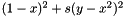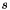## Apophenia

Optimization

This section includes some notes on the maximum likelihood routine. As in the section on writing models above, if a model has a `p` or `log_likelihood` method but no `estimate` method, then calling `apop_estimate(your_data, your_model)` executes the default estimation routine of maximum likelihood.

If you are a not a statistician, then there are a few things you will need to keep in mind:

• Physicists, pure mathematicians, and the GSL minimize; economists, statisticians, and Apophenia maximize. If you are doing a minimization, be sure that your function returns minus the objective function's value.
• The overall setup is about estimating the parameters of a model with data. The user provides a data set and an unparameterized model, and the system tries parameterized models until one of them is found to be optimal. The data is fixed. The optimization tries a series of parameterized models, searching for the one that is most likely. In a non-stats setting, you may have `NULL` data.
• Because the unit of analysis is a parameterized model, not just parameters, you need to have an apop_model wrapping your objective function.

This example, to be discussed in detail below, optimizes Rosenbrock's banana function,, where the scaling factoris fixed ahead of time, say at 100.

#include <apop.h>
typedef struct {
double scaling;
long double banana (double *params, coeff_struct *in){
return (gsl_pow_2(1-params)
+ in->scaling*gsl_pow_2(params-gsl_pow_2(params)));
}
long double ll (apop_data *d, apop_model *in){
return - banana(in->parameters->vector->data, in->more);
}
int main(){
coeff_struct co = {.scaling=100};
apop_model *b = &(apop_model) {"¡Bananas!", .log_likelihood= ll,
.vsize=2, .more = &co, .more_size=sizeof(coeff_struct)};
apop_model *e1 = apop_estimate(NULL, b);
//for printing the path below
apop_data *bfgs_path = NULL;
Apop_settings_set(b, apop_mle, path, &bfgs_path);
Apop_settings_set(b, apop_mle, method, "BFGS cg");
apop_model *e2 = apop_estimate(NULL, b);
apop_data_show(bfgs_path);
gsl_vector *one = apop_vector_fill(gsl_vector_alloc(2), 1, 1);
assert(apop_vector_distance(e1->parameters->vector, one) < 1e-2);
assert(apop_vector_distance(e2->parameters->vector, one) < 1e-2);
}

The `banana` function returns a single number to be minimized. You will need to write an apop_model to send to the optimizer, which is a two step process: write a log likelihood function wrapping the real objective function (`ll`), and a model that uses that log likelihood (`b`).

• The `.vsize=2` part of the declaration of `b` on the second line of `main()` specified that the model's parameters are a vector of size two. That is, the list of `double`s to send to `banana` is set in `in->parameters->vector->data`.
• The `more` element of the apop_model structure is designed to hold any arbitrary structure of size `more_size`, which is useful for models that require additional constants or other settings, like the `coeff_struct` here. See Writing new settings groups for more on handling model settings.
• Statisticians want the covariance and basic tests about the parameters. This line shuts off all auxiliary calculations:
See the documentation for apop_parts_wanted_settings for details about how this works. It can also offer quite the speedup: especially for high-dimensional problems, finding the covariance matrix without any additional information can take dozens of evaluations of the objective function for each evaluation that is part of the search itself.
• MLEs have an especially large number of parameter tweaks that could be made; see the apop_mle_settings page.
• As a useful diagnostic, you can add a `NULL` apop_data set to the MLE settings in the `.path` slot, and it will be allocated and filled with the sequence of points tried by the optimizer.
• The program has some extras above and beyond the necessary: it uses two methods (notice how easy it is to re-run an estimation with an alternate method, but the syntax for modifying a setting differs from the initialization syntax) and checks that the results are accurate.

# Setting Constraints

The problem is that the parameters of a function must not take on certain values, either because the function is undefined for those values or because parameters with certain values would not fit the real-world problem.

If you give the optimizer an unconstrained likelihood function plus a separate constraint function, apop_maximum_likelihood will combine them to a function that is continuous at the constraint boundary, but which is guaranteed to never have an optimum outside of the constraint.

A constraint function must do three things:

• If the constraint does not bind (i.e. the parameter values are OK), then it must return zero.
• If the constraint does bind, it must return a penalty, that indicates how far off the parameter is from meeting the constraint.
• If the constraint does bind, it must set a return vector that the likelihood function can take as a valid input. The penalty at this returned value must be zero.

The idea is that if the constraint returns zero, the log likelihood function will return the log likelihood as usual, and if not, it will return the log likelihood at the constraint's return vector minus the penalty. To give a concrete example, here is a constraint function that will ensure that both parameters of a two-dimensional input are both greater than zero, and that their sum is greater than two. As with the constraints for many of the models that ship with Apophenia, it is a wrapper for apop_linear_constraint.

static long double greater_than_zero_constraint(apop_data *data, apop_model *v){
static apop_data *constraint = NULL;
if (!constraint) constraint= apop_data_falloc((3,3,2), 0, 1, 0, //0 < 1x + 0y
0, 0, 1, //0 < 0x + 1y
2, 1, 1); //2 < 1x + 1y
return apop_linear_constraint(v->parameters->vector, constraint, 1e-3);
}

# Notes on simulated annealing

For convex optimizations, methods like conjugate gradient search work well, and for relatively smooth optimizations, the Nelder-Mead simplex algorithm is a good choice. For situations where the surface being searched may have several local optima and be otherwise badly behaved, there is simulated annealing.

Simulated annealing is a controlled random walk. As with the other methods, the system tries a new point, and if it is better, switches. Initially, the system is allowed to make large jumps, and then with each iteration, the jumps get smaller, eventually converging. Also, there is some decreasing probability that if the new point is less likely, it will still be chosen. Simulated annealing is best for situations where there may be multiple local optima. Early in the random walk, the system can readily jump from one to another; later it will fine-tune its way toward the optimum. The number of points tested is determined by the parameters of the simulated colling program, not the values returned by the likelihood function. If you know your function is globally convex (as are most standard probability functions), then this method is overkill.